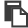【单选题】某气相 1-1 级平行反应 ,其指前因子 k 0,1 = k 0,2 ,活化能 E 1 ≠ E 2 ,但均与温度无关。现测得 298 K 时 k 1 / k 2 =100 ,则 754 K 时 k 1 / k 2 的值为
A.
2500
B.
2.5
C.
6.2
D.手机使用分享复制链接新浪微博分享QQ微信扫一扫反馈复制

【单选题】在平行反应中,开始时无任何产物,并且各平行反应级数相同,则在反应的任一时刻,各产物浓度之比为( )。
A.

B.

C.

D.

【单选题】如果平行反应A P(主)
A.
(副)，
B.

C.

D.

E.

F.

【简答题】平行反应各个反应的产物量之比等于其()。平行反应的选择性为一般可采用改变反应()或添加()等方法改变某平行反应的选择性,以利于更多得到所期望的产物。对于级数相同的平行反应,其产物浓度之比等于(),而与反应物的()和()无关。
【判断题】在平行反应中,若E1
A.

B.

【多选题】设有如下的平行反应： 对比选择性： 下列对于平行反应的对比选择性说法正确的有（    ）。
A.

B.

C.

D.

【单选题】下面描述的平行反应的特点,哪一点是不正确的?
A.
kA和kB比值不随温度而改变
B.

C.

D.

【判断题】内扩散的影响使平行反应中级数高者选择性高。
A.

B.

【单选题】关于平行反应 特点的描述,哪一点是不正确的?
A.
k 1 和k 2 的比值不随温度而变
B.

C.

D.

【单选题】对于平行反应，若主反应级数低于副反应级数，则宜选用（）
A.

B.

C.

【单选题】关于平行反应的选择性,下列说法正确的是( )。
A.

B.

C.

D.

【单选题】平行反应模型理论（PRM）是谁在1970年提出的？
A.
Leventhal
B.
Hovland
C.
Witte
D.
Rogers
【单选题】两个都是一级反应的 平行反应 :下列说法错误的是
A.
k 总 = k 1 + k 2
B.
E 总 = E 1 + E 2
C.
K 1 / K 2 = [D] / [H]
【单选题】描述平行反应的特点时,那一点是不正确的 。
A.
k 1 和k 2 的比值不随温度而变
B.

C.

D.

【单选题】对于平行反应A→R,A→S,活化能E1
A.

B.

C.

D.

【单选题】1. 物质 A 发生两个一级平行反应，，设两反应的指前因子相近且与温度无关，若 E1> E2，则有： ( )
A.
k1> k2
B.
k2 > k1
C.
k2= k1
D.

【单选题】若有一平行反应，,,E1,E2分别为主副反应活化能，选择性随温度上升而减小，则可以推断（ ）。
A.
E1<E2
B.
E1=E2
C.
E1>E2
D.

【单选题】下述结论对同级平行反应不适合的是( )。
A.

B.

C.

D.

【判断题】平行反应总的反应速率等于所有平行反应速率之和。
A.

B.

【单选题】平行反应A→B(k1),A→D(k2)的描述,不正确的是
A.
k1和k2的比值随温度而变
B.

C.

D.

【单选题】对于平行反应,若主反应级数高于副反应级数,则宜选用( )
A.

B.

C.

D.
A、B、C 均可
【判断题】对于平行反应，反应物消耗总速率为各平行反应消耗速率之和。
A.

B.

【单选题】下面关于平行反应的描述错误的是
A.

B.

C.

D.

【单选题】下面描述的平行反应(A B, A C)的特点,哪一点是不正确的?
A.
kB和kC比值不随温度而改
B.

C.

D.

【单选题】下面不属于平行反应特点的是
A.

B.

C.

D.

【单选题】对于平行反应,若主反应级数低于副反应级数,则宜选用( )
A.

B.

C.

D.

【单选题】下述结论对平行反应不适合的是
A.

B.

C.

D.

【判断题】内扩散的影响使平行反应中级数高者选择性高。
A.

B.

【单选题】下述关于平行反应说法不正确的是
A.

B.

C.

D.

【单选题】有两个都是一级的平行反应 下列哪个关系式是错误的?
A.
k 总 = k 1 + k 2
B.
C.
D.
E 总 = E 1 + E 2
【单选题】两一级平行反应A→B，A→C，下列结论不正确的是（ ）。
A.
k 总 = k 1+ k 2
B.
k 1 / k 2= c B/ c C
C.
E 总 = E 1+ E 2
D.
t 1/2 =0.693/( k 1+ k 2)
【单选题】级数相同的平行反应,其反应速率之比为常数,与( )无关。
A.

B.

C.

D.

【单选题】下面不属于平行反应特点的是
A.

B.

C.

D.

【多选题】设有如下的平行反应： 对比选择性： 下列对于平行反应的对比选择性说法正确的有（    ）。
A.

B.

C.

D.

【简答题】平行反应属于( );
【多选题】设有如下的平行反应： 【图片】对比选择性： 【图片】 下列对于平行反应的对比选择性说法正确的有（ ）。
A.

B.

C.

D.

【多选题】两个一级平行反应 A B , AC ,下列哪个结论是正确的:
A.
k 总 = k 1 + k 2
B.
k 1 / k 2 = [B]/[C]
C.
E 总 = E 1 + E 2
D.
t = 0.693/( k 1 + k 2 )
【判断题】平行反应总反应速率常数，等于同时进行的各个反应速率常数之和
A.
B.
【判断题】平行反应的总反应速率等于各平行反应速率之和。( )
A.

B.

【单选题】返混对主反应级数大于副反应级数的平行反应是（ ）的。
A.

B.

C.

D.

【单选题】下面描述的平行反应 的特点,哪一点是不正确的 ?
A.
k B 和 k C 比值不随温度而改变
B.

C.

D.

【单选题】有两个都是一级的平行反应 下列哪个关系式是错误的? ( )
A.
k总=k1+k2;
B.
E总=E1+E2;
C.
;
D.
;
E.

【单选题】物质 A 发生两个一级平行反应，，设两反应的指前因子相近且与温度无关，若 E1> E2，则有： ( )
A.

B.

k2= k1

C.

k1> k2

D.

k2 > k1

【单选题】两个一级平行反应 下列哪个结论是不正确的：（）
A.
k（总）=k1+ k2
B.
k1/k2= [B]/[C]
C.
E（总）= E1+ E2
D.
t（1/2）= 0.693/（k1+ k2）
【判断题】平行反应的总速率等于各平行反应速率之和。反应物消耗速率主要取决最慢的分支反应。
A.

B.

【单选题】平行反应属于( )
A.

B.

C.

D.

【单选题】平行反应模型理论(PRM)是谁在1970年提出的?
A.
Rogers
B.
Hovland
C.
Leventhal
D.
Witte
【单选题】对于平行反应，若主反应级数高于副反应级数，则宜选用（）
A.

B.

C.

D.
D、B、C均可
【判断题】平行反应中,反应物的消耗速率由平行各反应共同决定。
A.

B.

【多选题】下述结论对平行反应适合的是
A.

B.

C.

D.

【简答题】下列属于平行反应的是( )。A. B. B. C. C. D. D.© 2019 pipixue.com 京ICP备20000060号-6 京公网安备 :11010802030703